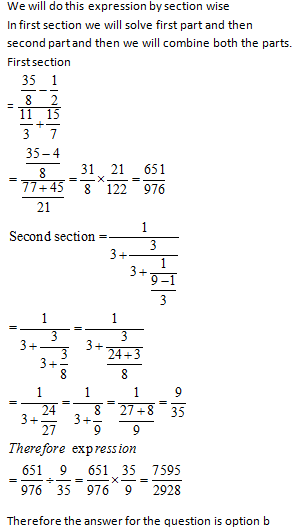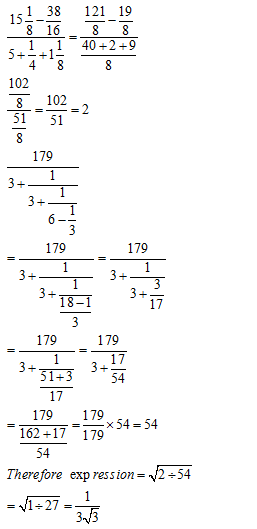• This is an assessment test.
• These tests focus on the basics of Maths and are meant to indicate your preparation level for the subject.
• Kindly take the tests in this series with a pre-defined schedule.

## Basic Maths: Test 28

Congratulations - you have completed Basic Maths: Test 28. You scored %%SCORE%% out of %%TOTAL%%. You correct answer percentage: %%PERCENTAGE%% . Your performance has been rated as %%RATING%%
 Question 1
$\frac{4\frac{3}{8}-\frac{1}{2}}{3\frac{2}{3}+2\frac{1}{7}}\div \frac{1}{3+\frac{3}{3+\frac{1}{3-\frac{1}{3}}}}$
is equal to
 A $\frac{7699}{2928}$ B $\frac{7595}{2928}$ C $\frac{75}{2928}$ D $\frac{759}{2928}$
Question 1 Explanation:Question 2
$If\,\,{{\left[ 4-\frac{5}{3-\frac{1}{\frac{5}{9}+\frac{1}{2+\frac{1}{4}}}} \right]}^{th}}$
part of a journey takes 10 minutes, then to compete 6/5th of that journey, it will take
 A 3 minutes B 13 minutes C 24 minutes D 8 minutes
Question 2 Explanation:
We are given by a fraction which is a part of any Distance
First we will solve that fraction part then we will proceed for the further question
$\displaystyle \begin{array}{l}4-\frac{5}{3-\frac{1}{\frac{5}{9}+\frac{1}{\frac{9}{4}}}}=4-\frac{5}{3-\frac{1}{\frac{5}{9}+\frac{4}{9}}}\\=4-\frac{5}{3-1}=4-\frac{5}{2}\\=\frac{8-5}{2}\\=\frac{3}{2}\\Time\,\,\,taken\,\,in\,\,\,completing\,\,\,=10\,\,\min utes\\Therefore\,\,\,time\,\,taken\,\,\,in\,\,\,completing\,\,\frac{6}{5}\,\,part\\=10\times \frac{2}{3}\times \frac{6}{5}\\=8\,\,Minutes\end{array}$
 Question 3
$\left( 1001\frac{1001}{1000}\times 7 \right)$
is equals to
 A $6998\frac{1}{1000}$ B $7008\frac{7}{1000}$ C $7003\frac{1}{1000}$ D $7014\frac{7}{1000}$
Question 3 Explanation:
$\begin{array}{l}1001\frac{1001}{1000}\times 7\\=\left( 1001+\frac{1001}{1000} \right)\times 7\\=7007+\frac{7007}{1000}\\=7007+7\frac{7}{1000}\\=7014\frac{7}{1000}\end{array}$
 Question 4
$\sqrt{\frac{15\frac{1}{8}-\frac{38}{16}}{5+\frac{1}{4}+1\frac{1}{8}}\div \frac{179}{3+\frac{1}{3+\frac{1}{6-\frac{1}{3}}}}}$
is equal to
 A $\frac{1}{3\sqrt{3}}$ B $3\sqrt{3}$ C $3$ D $\sqrt{3}$
Question 4 Explanation:Question 5
The sum ‘9+…..+19’ is equal to:
 A 150 B 160 C 154 D 164
Question 5 Explanation:
$\begin{array}{l}1+2+3+.....+n=\frac{n\left( n+1 \right)}{2}\\Therefore\,9+10+11+....+19\\=\left( 1+2+3+.....+19 \right)-\left( 1+2+3....+8 \right)\\=\frac{19\left( 19+1 \right)}{2}-\frac{8\left( 8+1 \right)}{2}=190-36=154\end{array}$
Once you are finished, click the button below. Any items you have not completed will be marked incorrect.
There are 5 questions to complete.
 ← List →